Anúncio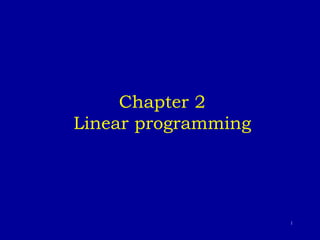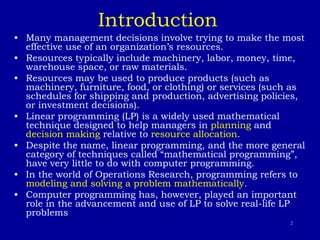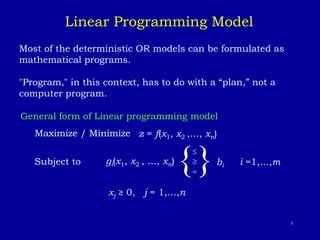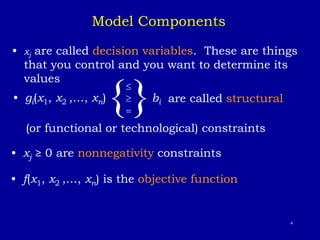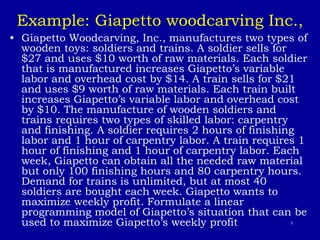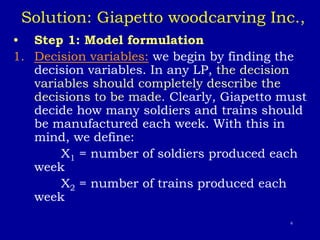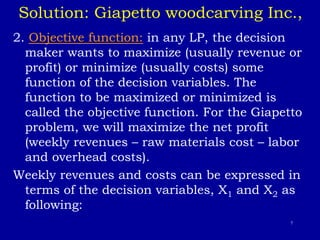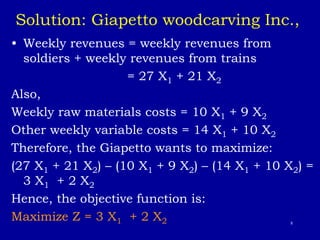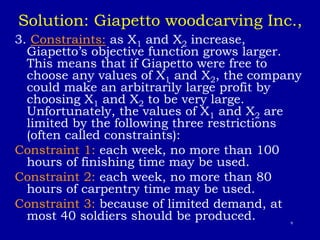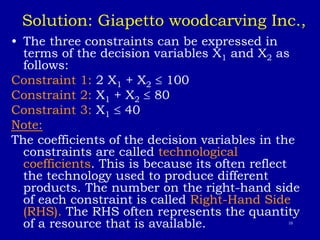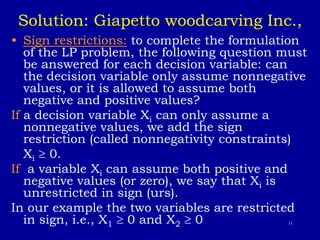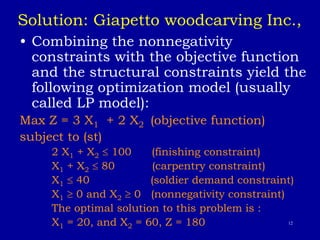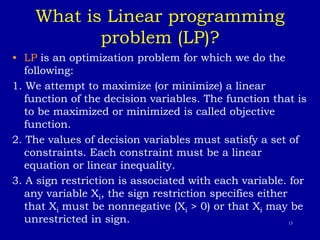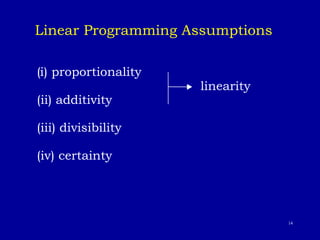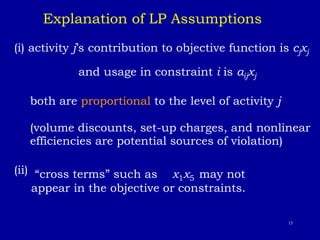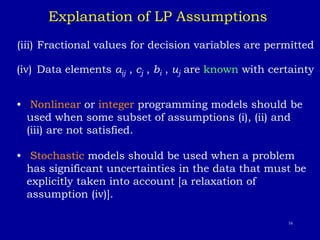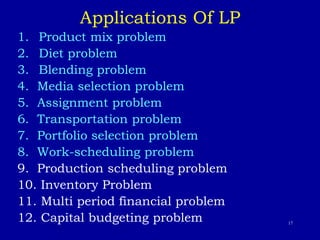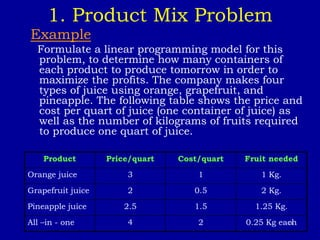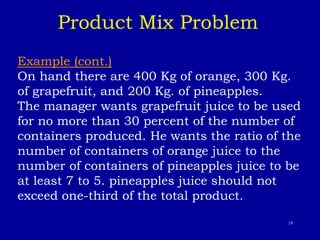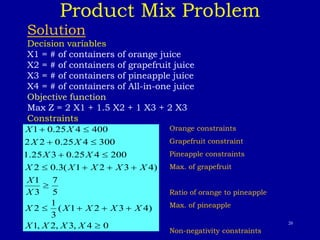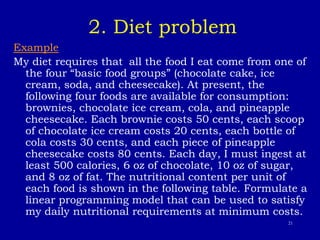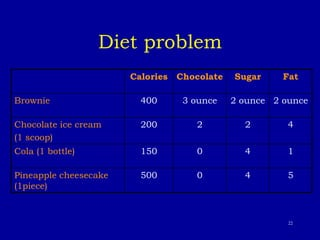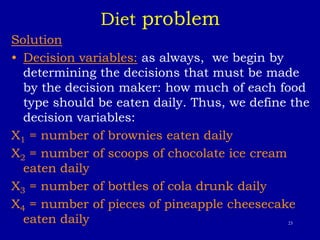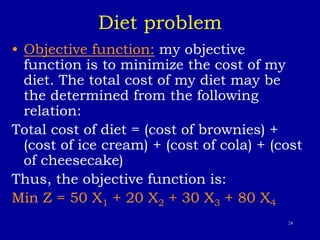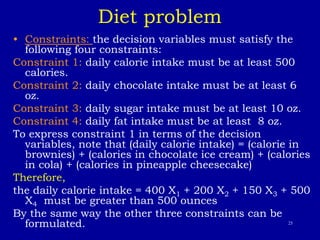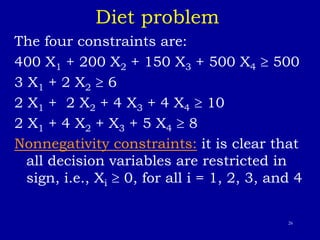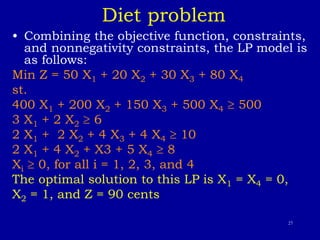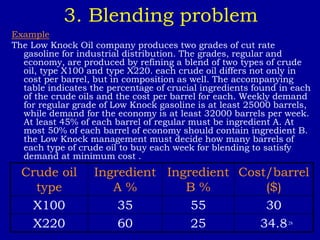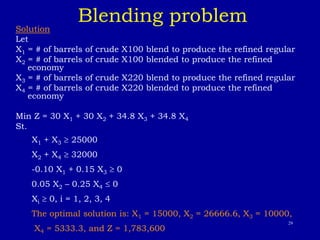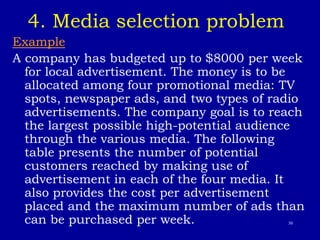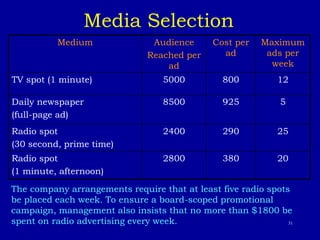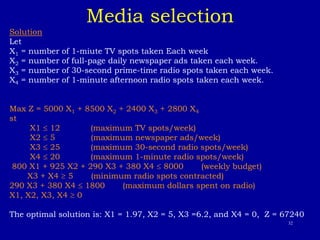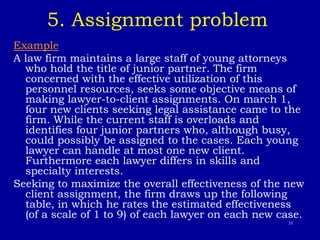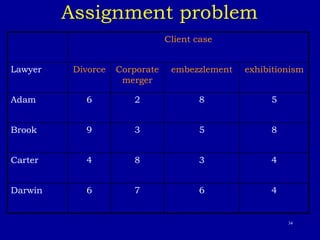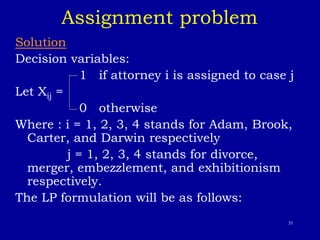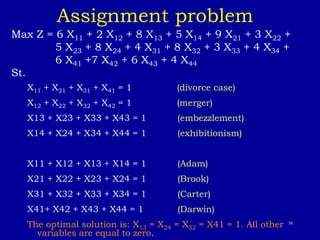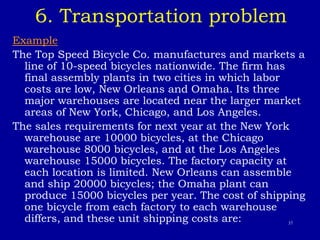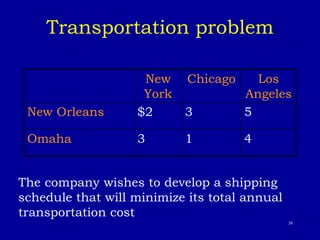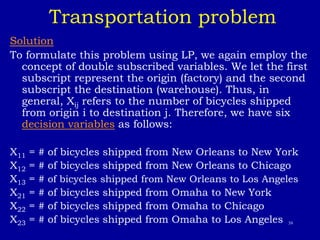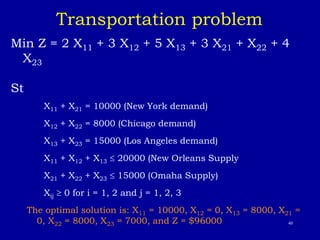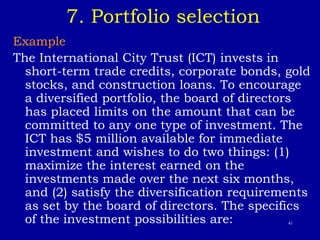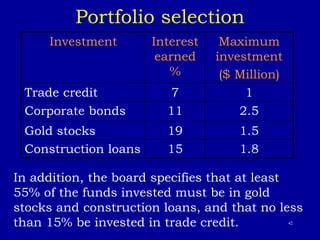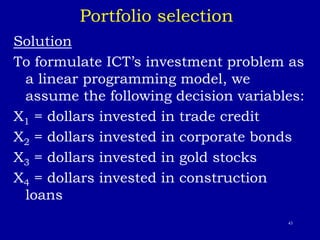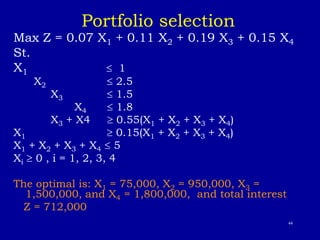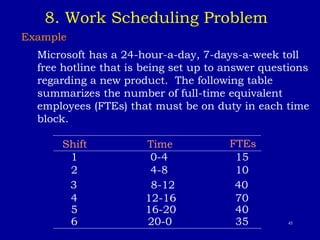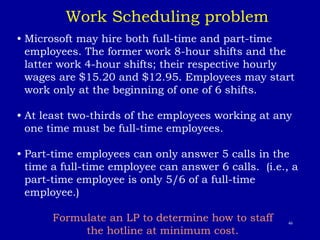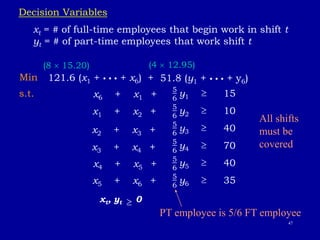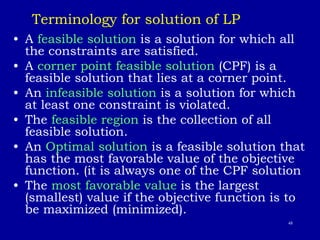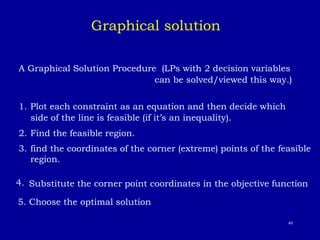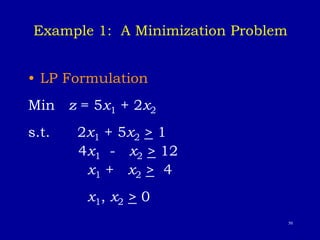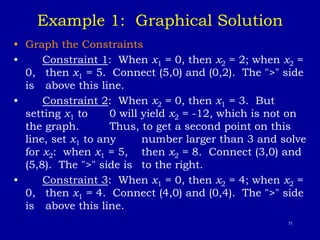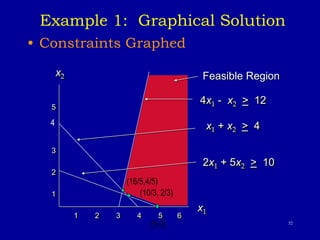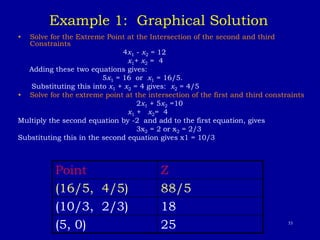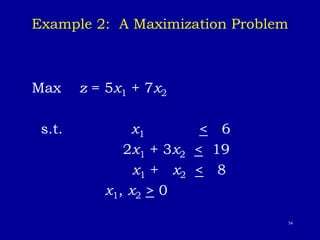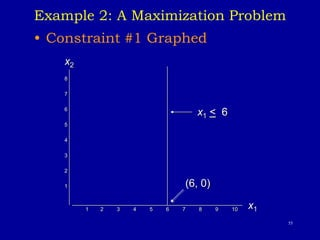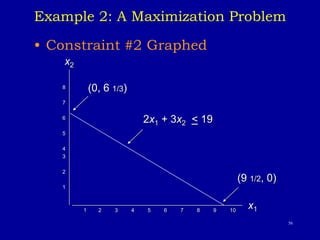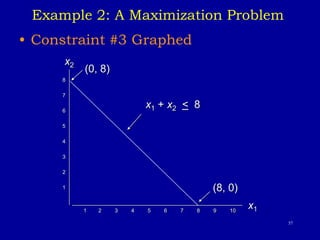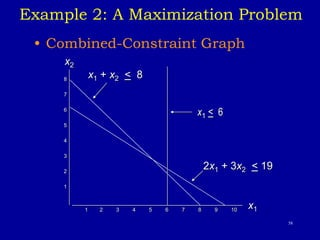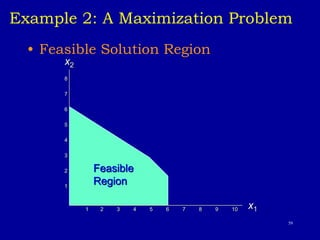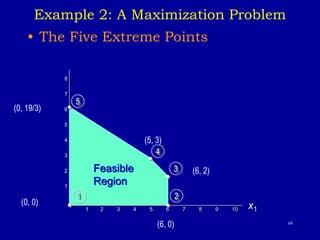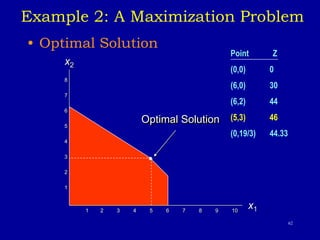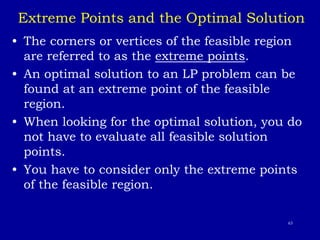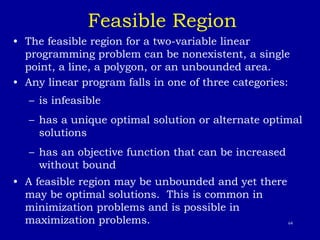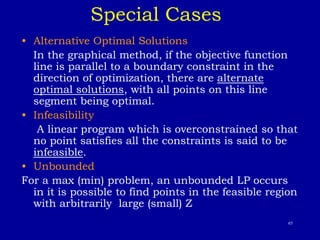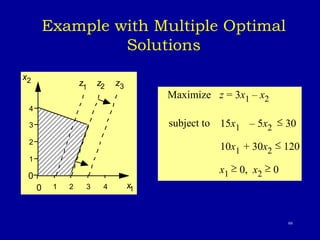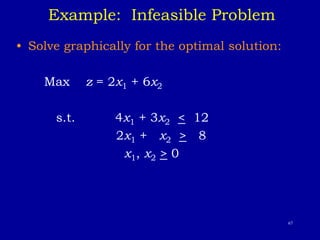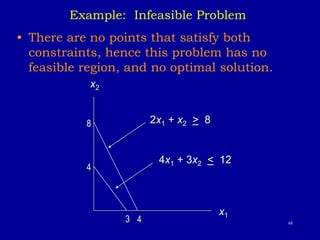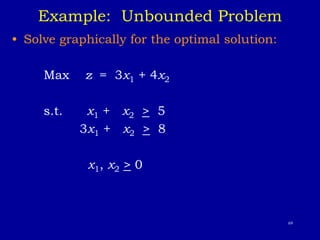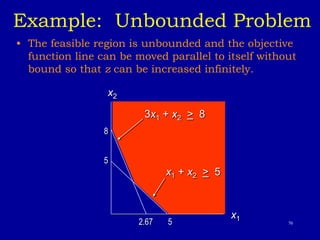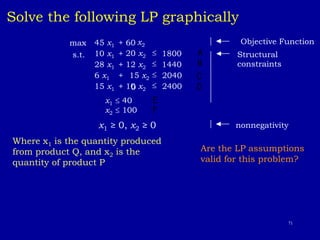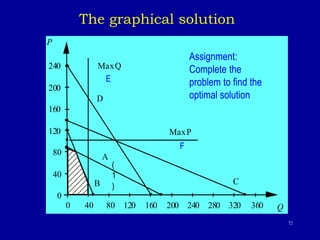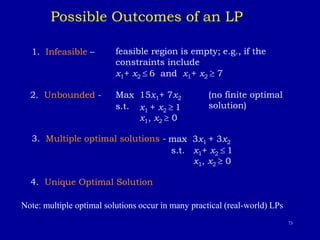1 de 73
Anúncio

### lp 2.ppt

1. 1 Chapter 2 Linear programming
2. 2 Introduction • Many management decisions involve trying to make the most effective use of an organization’s resources. • Resources typically include machinery, labor, money, time, warehouse space, or raw materials. • Resources may be used to produce products (such as machinery, furniture, food, or clothing) or services (such as schedules for shipping and production, advertising policies, or investment decisions). • Linear programming (LP) is a widely used mathematical technique designed to help managers in planning and decision making relative to resource allocation. • Despite the name, linear programming, and the more general category of techniques called “mathematical programming”, have very little to do with computer programming. • In the world of Operations Research, programming refers to modeling and solving a problem mathematically. • Computer programming has, however, played an important role in the advancement and use of LP to solve real-life LP problems
3. 3 Most of the deterministic OR models can be formulated as mathematical programs. "Program," in this context, has to do with a “plan,” not a computer program. General form of Linear programming model Maximize / Minimize z = f(x1, x2 ,…, xn) Subject to {  = } bi i =1,…,m xj ≥ 0, j = 1,…,n Linear Programming Model gi(x1, x2 , …, xn)
4. 4 • xj are called decision variables. These are things that you control and you want to determine its values {  = }bi are called structural (or functional or technological) constraints • xj ≥ 0 are nonnegativity constraints Model Components • f(x1, x2 ,…, xn) is the objective function • gi(x1, x2 ,…, xn)
5. 5 Example: Giapetto woodcarving Inc., • Giapetto Woodcarving, Inc., manufactures two types of wooden toys: soldiers and trains. A soldier sells for \$27 and uses \$10 worth of raw materials. Each soldier that is manufactured increases Giapetto’s variable labor and overhead cost by \$14. A train sells for \$21 and uses \$9 worth of raw materials. Each train built increases Giapetto’s variable labor and overhead cost by \$10. The manufacture of wooden soldiers and trains requires two types of skilled labor: carpentry and finishing. A soldier requires 2 hours of finishing labor and 1 hour of carpentry labor. A train requires 1 hour of finishing and 1 hour of carpentry labor. Each week, Giapetto can obtain all the needed raw material but only 100 finishing hours and 80 carpentry hours. Demand for trains is unlimited, but at most 40 soldiers are bought each week. Giapetto wants to maximize weekly profit. Formulate a linear programming model of Giapetto’s situation that can be used to maximize Giapetto’s weekly profit
6. 6 Solution: Giapetto woodcarving Inc., • Step 1: Model formulation 1. Decision variables: we begin by finding the decision variables. In any LP, the decision variables should completely describe the decisions to be made. Clearly, Giapetto must decide how many soldiers and trains should be manufactured each week. With this in mind, we define: X1 = number of soldiers produced each week X2 = number of trains produced each week
7. 7 Solution: Giapetto woodcarving Inc., 2. Objective function: in any LP, the decision maker wants to maximize (usually revenue or profit) or minimize (usually costs) some function of the decision variables. The function to be maximized or minimized is called the objective function. For the Giapetto problem, we will maximize the net profit (weekly revenues – raw materials cost – labor and overhead costs). Weekly revenues and costs can be expressed in terms of the decision variables, X1 and X2 as following:
8. 8 Solution: Giapetto woodcarving Inc., • Weekly revenues = weekly revenues from soldiers + weekly revenues from trains = 27 X1 + 21 X2 Also, Weekly raw materials costs = 10 X1 + 9 X2 Other weekly variable costs = 14 X1 + 10 X2 Therefore, the Giapetto wants to maximize: (27 X1 + 21 X2) – (10 X1 + 9 X2) – (14 X1 + 10 X2) = 3 X1 + 2 X2 Hence, the objective function is: Maximize Z = 3 X1 + 2 X2
9. 9 Solution: Giapetto woodcarving Inc., 3. Constraints: as X1 and X2 increase, Giapetto’s objective function grows larger. This means that if Giapetto were free to choose any values of X1 and X2, the company could make an arbitrarily large profit by choosing X1 and X2 to be very large. Unfortunately, the values of X1 and X2 are limited by the following three restrictions (often called constraints): Constraint 1: each week, no more than 100 hours of finishing time may be used. Constraint 2: each week, no more than 80 hours of carpentry time may be used. Constraint 3: because of limited demand, at most 40 soldiers should be produced.
10. 10 Solution: Giapetto woodcarving Inc., • The three constraints can be expressed in terms of the decision variables X1 and X2 as follows: Constraint 1: 2 X1 + X2  100 Constraint 2: X1 + X2  80 Constraint 3: X1  40 Note: The coefficients of the decision variables in the constraints are called technological coefficients. This is because its often reflect the technology used to produce different products. The number on the right-hand side of each constraint is called Right-Hand Side (RHS). The RHS often represents the quantity of a resource that is available.
11. 11 Solution: Giapetto woodcarving Inc., • Sign restrictions: to complete the formulation of the LP problem, the following question must be answered for each decision variable: can the decision variable only assume nonnegative values, or it is allowed to assume both negative and positive values? If a decision variable Xi can only assume a nonnegative values, we add the sign restriction (called nonnegativity constraints) Xi  0. If a variable Xi can assume both positive and negative values (or zero), we say that Xi is unrestricted in sign (urs). In our example the two variables are restricted in sign, i.e., X1  0 and X2  0
12. 12 Solution: Giapetto woodcarving Inc., • Combining the nonnegativity constraints with the objective function and the structural constraints yield the following optimization model (usually called LP model): Max Z = 3 X1 + 2 X2 (objective function) subject to (st) 2 X1 + X2  100 (finishing constraint) X1 + X2  80 (carpentry constraint) X1  40 (soldier demand constraint) X1  0 and X2  0 (nonnegativity constraint) The optimal solution to this problem is : X1 = 20, and X2 = 60, Z = 180
13. 13 What is Linear programming problem (LP)? • LP is an optimization problem for which we do the following: 1. We attempt to maximize (or minimize) a linear function of the decision variables. The function that is to be maximized or minimized is called objective function. 2. The values of decision variables must satisfy a set of constraints. Each constraint must be a linear equation or linear inequality. 3. A sign restriction is associated with each variable. for any variable Xi, the sign restriction specifies either that Xi must be nonnegative (Xi > 0) or that Xi may be unrestricted in sign.
14. 14 Linear Programming Assumptions (i) proportionality (ii) additivity linearity (iii) divisibility (iv) certainty
15. 15 (i) activity j’s contribution to objective function is cjxj and usage in constraint i is aijxj both are proportional to the level of activity j (volume discounts, set-up charges, and nonlinear efficiencies are potential sources of violation) (ii) “cross terms” such as x1x5 may not appear in the objective or constraints. Explanation of LP Assumptions
16. 16 (iii) Fractional values for decision variables are permitted (iv) Data elements aij , cj , bi , uj are known with certainty • Nonlinear or integer programming models should be used when some subset of assumptions (i), (ii) and (iii) are not satisfied. • Stochastic models should be used when a problem has significant uncertainties in the data that must be explicitly taken into account [a relaxation of assumption (iv)]. Explanation of LP Assumptions
17. 17 Applications Of LP 1. Product mix problem 2. Diet problem 3. Blending problem 4. Media selection problem 5. Assignment problem 6. Transportation problem 7. Portfolio selection problem 8. Work-scheduling problem 9. Production scheduling problem 10. Inventory Problem 11. Multi period financial problem 12. Capital budgeting problem
18. 18 1. Product Mix Problem Example Formulate a linear programming model for this problem, to determine how many containers of each product to produce tomorrow in order to maximize the profits. The company makes four types of juice using orange, grapefruit, and pineapple. The following table shows the price and cost per quart of juice (one container of juice) as well as the number of kilograms of fruits required to produce one quart of juice. Product Price/quart Cost/quart Fruit needed Orange juice 3 1 1 Kg. Grapefruit juice 2 0.5 2 Kg. Pineapple juice 2.5 1.5 1.25 Kg. All –in - one 4 2 0.25 Kg each
19. 19 Example (cont.) On hand there are 400 Kg of orange, 300 Kg. of grapefruit, and 200 Kg. of pineapples. The manager wants grapefruit juice to be used for no more than 30 percent of the number of containers produced. He wants the ratio of the number of containers of orange juice to the number of containers of pineapples juice to be at least 7 to 5. pineapples juice should not exceed one-third of the total product. Product Mix Problem
20. 20 Product Mix Problem Solution Decision variables X1 = # of containers of orange juice X2 = # of containers of grapefruit juice X3 = # of containers of pineapple juice X4 = # of containers of All-in-one juice Objective function Max Z = 2 X1 + 1.5 X2 + 1 X3 + 2 X3 Constraints 0 4 , 3 , 2 , 1 ) 4 3 2 1 ( 3 1 2 5 7 3 1 ) 4 3 2 1 ( 3 . 0 2 200 4 25 . 0 3 25 . 1 300 4 25 . 0 2 2 400 4 25 . 0 1                 X X X X X X X X X X X X X X X X X X X X X X Orange constraints Grapefruit constraint Pineapple constraints Max. of grapefruit Ratio of orange to pineapple Max. of pineapple Non-negativity constraints
21. 21 2. Diet problem Example My diet requires that all the food I eat come from one of the four “basic food groups” (chocolate cake, ice cream, soda, and cheesecake). At present, the following four foods are available for consumption: brownies, chocolate ice cream, cola, and pineapple cheesecake. Each brownie costs 50 cents, each scoop of chocolate ice cream costs 20 cents, each bottle of cola costs 30 cents, and each piece of pineapple cheesecake costs 80 cents. Each day, I must ingest at least 500 calories, 6 oz of chocolate, 10 oz of sugar, and 8 oz of fat. The nutritional content per unit of each food is shown in the following table. Formulate a linear programming model that can be used to satisfy my daily nutritional requirements at minimum costs.
22. 22 Diet problem Calories Chocolate Sugar Fat Brownie 400 3 ounce 2 ounce 2 ounce Chocolate ice cream (1 scoop) 200 2 2 4 Cola (1 bottle) 150 0 4 1 Pineapple cheesecake (1piece) 500 0 4 5
23. 23 Diet problem Solution • Decision variables: as always, we begin by determining the decisions that must be made by the decision maker: how much of each food type should be eaten daily. Thus, we define the decision variables: X1 = number of brownies eaten daily X2 = number of scoops of chocolate ice cream eaten daily X3 = number of bottles of cola drunk daily X4 = number of pieces of pineapple cheesecake eaten daily
24. 24 Diet problem • Objective function: my objective function is to minimize the cost of my diet. The total cost of my diet may be the determined from the following relation: Total cost of diet = (cost of brownies) + (cost of ice cream) + (cost of cola) + (cost of cheesecake) Thus, the objective function is: Min Z = 50 X1 + 20 X2 + 30 X3 + 80 X4
25. 25 Diet problem • Constraints: the decision variables must satisfy the following four constraints: Constraint 1: daily calorie intake must be at least 500 calories. Constraint 2: daily chocolate intake must be at least 6 oz. Constraint 3: daily sugar intake must be at least 10 oz. Constraint 4: daily fat intake must be at least 8 oz. To express constraint 1 in terms of the decision variables, note that (daily calorie intake) = (calorie in brownies) + (calories in chocolate ice cream) + (calories in cola) + (calories in pineapple cheesecake) Therefore, the daily calorie intake = 400 X1 + 200 X2 + 150 X3 + 500 X4 must be greater than 500 ounces By the same way the other three constraints can be formulated.
26. 26 Diet problem The four constraints are: 400 X1 + 200 X2 + 150 X3 + 500 X4  500 3 X1 + 2 X2  6 2 X1 + 2 X2 + 4 X3 + 4 X4  10 2 X1 + 4 X2 + X3 + 5 X4  8 Nonnegativity constraints: it is clear that all decision variables are restricted in sign, i.e., Xi  0, for all i = 1, 2, 3, and 4
27. 27 Diet problem • Combining the objective function, constraints, and nonnegativity constraints, the LP model is as follows: Min Z = 50 X1 + 20 X2 + 30 X3 + 80 X4 st. 400 X1 + 200 X2 + 150 X3 + 500 X4  500 3 X1 + 2 X2  6 2 X1 + 2 X2 + 4 X3 + 4 X4  10 2 X1 + 4 X2 + X3 + 5 X4  8 Xi  0, for all i = 1, 2, 3, and 4 The optimal solution to this LP is X1 = X4 = 0, X2 = 1, and Z = 90 cents
28. 28 3. Blending problem Example The Low Knock Oil company produces two grades of cut rate gasoline for industrial distribution. The grades, regular and economy, are produced by refining a blend of two types of crude oil, type X100 and type X220. each crude oil differs not only in cost per barrel, but in composition as well. The accompanying table indicates the percentage of crucial ingredients found in each of the crude oils and the cost per barrel for each. Weekly demand for regular grade of Low Knock gasoline is at least 25000 barrels, while demand for the economy is at least 32000 barrels per week. At least 45% of each barrel of regular must be ingredient A. At most 50% of each barrel of economy should contain ingredient B. the Low Knock management must decide how many barrels of each type of crude oil to buy each week for blending to satisfy demand at minimum cost . Crude oil type Ingredient A % Ingredient B % Cost/barrel (\$) X100 35 55 30 X220 60 25 34.8
29. 29 Blending problem Solution Let X1 = # of barrels of crude X100 blend to produce the refined regular X2 = # of barrels of crude X100 blended to produce the refined economy X3 = # of barrels of crude X220 blend to produce the refined regular X4 = # of barrels of crude X220 blended to produce the refined economy Min Z = 30 X1 + 30 X2 + 34.8 X3 + 34.8 X4 St. X1 + X3  25000 X2 + X4  32000 -0.10 X1 + 0.15 X3  0 0.05 X2 – 0.25 X4  0 Xi  0, i = 1, 2, 3, 4 The optimal solution is: X1 = 15000, X2 = 26666.6, X3 = 10000, X4 = 5333.3, and Z = 1,783,600
31. 31 Media Selection Medium Audience Reached per ad Cost per ad Maximum ads per week TV spot (1 minute) 5000 800 12 Daily newspaper (full-page ad) 8500 925 5 Radio spot (30 second, prime time) 2400 290 25 Radio spot (1 minute, afternoon) 2800 380 20 The company arrangements require that at least five radio spots be placed each week. To ensure a board-scoped promotional campaign, management also insists that no more than \$1800 be spent on radio advertising every week.
32. 32 Media selection Solution Let X1 = number of 1-miute TV spots taken Each week X2 = number of full-page daily newspaper ads taken each week. X3 = number of 30-second prime-time radio spots taken each week. X4 = number of 1-minute afternoon radio spots taken each week. Max Z = 5000 X1 + 8500 X2 + 2400 X3 + 2800 X4 st X1  12 (maximum TV spots/week) X2  5 (maximum newspaper ads/week) X3  25 (maximum 30-second radio spots/week) X4  20 (maximum 1-minute radio spots/week) 800 X1 + 925 X2 + 290 X3 + 380 X4  8000 (weekly budget) X3 + X4  5 (minimum radio spots contracted) 290 X3 + 380 X4  1800 (maximum dollars spent on radio) X1, X2, X3, X4  0 The optimal solution is: X1 = 1.97, X2 = 5, X3 =6.2, and X4 = 0, Z = 67240
33. 33 5. Assignment problem Example A law firm maintains a large staff of young attorneys who hold the title of junior partner. The firm concerned with the effective utilization of this personnel resources, seeks some objective means of making lawyer-to-client assignments. On march 1, four new clients seeking legal assistance came to the firm. While the current staff is overloads and identifies four junior partners who, although busy, could possibly be assigned to the cases. Each young lawyer can handle at most one new client. Furthermore each lawyer differs in skills and specialty interests. Seeking to maximize the overall effectiveness of the new client assignment, the firm draws up the following table, in which he rates the estimated effectiveness (of a scale of 1 to 9) of each lawyer on each new case.
34. 34 Assignment problem Client case Lawyer Divorce Corporate merger embezzlement exhibitionism Adam 6 2 8 5 Brook 9 3 5 8 Carter 4 8 3 4 Darwin 6 7 6 4
35. 35 Assignment problem Solution Decision variables: 1 if attorney i is assigned to case j Let Xij = 0 otherwise Where : i = 1, 2, 3, 4 stands for Adam, Brook, Carter, and Darwin respectively j = 1, 2, 3, 4 stands for divorce, merger, embezzlement, and exhibitionism respectively. The LP formulation will be as follows:
36. 36 Assignment problem Max Z = 6 X11 + 2 X12 + 8 X13 + 5 X14 + 9 X21 + 3 X22 + 5 X23 + 8 X24 + 4 X31 + 8 X32 + 3 X33 + 4 X34 + 6 X41 +7 X42 + 6 X43 + 4 X44 St. X11 + X21 + X31 + X41 = 1 (divorce case) X12 + X22 + X32 + X42 = 1 (merger) X13 + X23 + X33 + X43 = 1 (embezzlement) X14 + X24 + X34 + X44 = 1 (exhibitionism) X11 + X12 + X13 + X14 = 1 (Adam) X21 + X22 + X23 + X24 = 1 (Brook) X31 + X32 + X33 + X34 = 1 (Carter) X41+ X42 + X43 + X44 = 1 (Darwin) The optimal solution is: X13 = X24 = X32 = X41 = 1. All other variables are equal to zero.
37. 37 6. Transportation problem Example The Top Speed Bicycle Co. manufactures and markets a line of 10-speed bicycles nationwide. The firm has final assembly plants in two cities in which labor costs are low, New Orleans and Omaha. Its three major warehouses are located near the larger market areas of New York, Chicago, and Los Angeles. The sales requirements for next year at the New York warehouse are 10000 bicycles, at the Chicago warehouse 8000 bicycles, and at the Los Angeles warehouse 15000 bicycles. The factory capacity at each location is limited. New Orleans can assemble and ship 20000 bicycles; the Omaha plant can produce 15000 bicycles per year. The cost of shipping one bicycle from each factory to each warehouse differs, and these unit shipping costs are:
38. 38 Transportation problem New York Chicago Los Angeles New Orleans \$2 3 5 Omaha 3 1 4 The company wishes to develop a shipping schedule that will minimize its total annual transportation cost
39. 39 Transportation problem Solution To formulate this problem using LP, we again employ the concept of double subscribed variables. We let the first subscript represent the origin (factory) and the second subscript the destination (warehouse). Thus, in general, Xij refers to the number of bicycles shipped from origin i to destination j. Therefore, we have six decision variables as follows: X11 = # of bicycles shipped from New Orleans to New York X12 = # of bicycles shipped from New Orleans to Chicago X13 = # of bicycles shipped from New Orleans to Los Angeles X21 = # of bicycles shipped from Omaha to New York X22 = # of bicycles shipped from Omaha to Chicago X23 = # of bicycles shipped from Omaha to Los Angeles
40. 40 Transportation problem Min Z = 2 X11 + 3 X12 + 5 X13 + 3 X21 + X22 + 4 X23 St X11 + X21 = 10000 (New York demand) X12 + X22 = 8000 (Chicago demand) X13 + X23 = 15000 (Los Angeles demand) X11 + X12 + X13  20000 (New Orleans Supply X21 + X22 + X23  15000 (Omaha Supply) Xij  0 for i = 1, 2 and j = 1, 2, 3 The optimal solution is: X11 = 10000, X12 = 0, X13 = 8000, X21 = 0, X22 = 8000, X23 = 7000, and Z = \$96000
41. 41 7. Portfolio selection Example The International City Trust (ICT) invests in short-term trade credits, corporate bonds, gold stocks, and construction loans. To encourage a diversified portfolio, the board of directors has placed limits on the amount that can be committed to any one type of investment. The ICT has \$5 million available for immediate investment and wishes to do two things: (1) maximize the interest earned on the investments made over the next six months, and (2) satisfy the diversification requirements as set by the board of directors. The specifics of the investment possibilities are:
42. 42 Portfolio selection Investment Interest earned % Maximum investment (\$ Million) Trade credit 7 1 Corporate bonds 11 2.5 Gold stocks 19 1.5 Construction loans 15 1.8 In addition, the board specifies that at least 55% of the funds invested must be in gold stocks and construction loans, and that no less than 15% be invested in trade credit.
43. 43 Portfolio selection Solution To formulate ICT’s investment problem as a linear programming model, we assume the following decision variables: X1 = dollars invested in trade credit X2 = dollars invested in corporate bonds X3 = dollars invested in gold stocks X4 = dollars invested in construction loans
44. 44 Portfolio selection Max Z = 0.07 X1 + 0.11 X2 + 0.19 X3 + 0.15 X4 St. X1  1 X2  2.5 X3  1.5 X4  1.8 X3 + X4  0.55(X1 + X2 + X3 + X4) X1  0.15(X1 + X2 + X3 + X4) X1 + X2 + X3 + X4  5 Xi  0 , i = 1, 2, 3, 4 The optimal is: X1 = 75,000, X2 = 950,000, X3 = 1,500,000, and X4 = 1,800,000, and total interest Z = 712,000
45. 45 8. Work Scheduling Problem Example Microsoft has a 24-hour-a-day, 7-days-a-week toll free hotline that is being set up to answer questions regarding a new product. The following table summarizes the number of full-time equivalent employees (FTEs) that must be on duty in each time block. Shift Time FTEs 1 0-4 15 2 4-8 10 3 8-12 40 4 12-16 70 5 16-20 40 6 20-0 35
46. 46 • Microsoft may hire both full-time and part-time employees. The former work 8-hour shifts and the latter work 4-hour shifts; their respective hourly wages are \$15.20 and \$12.95. Employees may start work only at the beginning of one of 6 shifts. • At least two-thirds of the employees working at any one time must be full-time employees. • Part-time employees can only answer 5 calls in the time a full-time employee can answer 6 calls. (i.e., a part-time employee is only 5/6 of a full-time employee.) Formulate an LP to determine how to staff the hotline at minimum cost. Work Scheduling problem
47. 47 Decision Variables xt = # of full-time employees that begin work in shift t yt = # of part-time employees that work shift t Min 121.6 (x1 + • • • + x6) + 51.8 (y1 + • • • + y6) s.t. 5 6 y1  15 5 6 y2  10 5 6 y3  40 5 6 y4  70 5 6 y5  40 5 6 y6  35 (8  15.20) (4  12.95) All shifts must be covered PT employee is 5/6 FT employee x6 + x1 + x1 + x2 + x2 + x3 + x3 + x4 + x4 + x5 + x5 + x6 + xt, yt 0 
48. 48 Terminology for solution of LP • A feasible solution is a solution for which all the constraints are satisfied. • A corner point feasible solution (CPF) is a feasible solution that lies at a corner point. • An infeasible solution is a solution for which at least one constraint is violated. • The feasible region is the collection of all feasible solution. • An Optimal solution is a feasible solution that has the most favorable value of the objective function. (it is always one of the CPF solution • The most favorable value is the largest (smallest) value if the objective function is to be maximized (minimized).
49. 49 Graphical solution A Graphical Solution Procedure (LPs with 2 decision variables can be solved/viewed this way.) 1. Plot each constraint as an equation and then decide which side of the line is feasible (if it’s an inequality). 2. Find the feasible region. 3. find the coordinates of the corner (extreme) points of the feasible region. 4. Substitute the corner point coordinates in the objective function 5. Choose the optimal solution
50. 50 Example 1: A Minimization Problem • LP Formulation Min z = 5x1 + 2x2 s.t. 2x1 + 5x2 > 1 4x1 - x2 > 12 x1 + x2 > 4 x1, x2 > 0
51. 51 Example 1: Graphical Solution • Graph the Constraints • Constraint 1: When x1 = 0, then x2 = 2; when x2 = 0, then x1 = 5. Connect (5,0) and (0,2). The ">" side is above this line. • Constraint 2: When x2 = 0, then x1 = 3. But setting x1 to 0 will yield x2 = -12, which is not on the graph. Thus, to get a second point on this line, set x1 to any number larger than 3 and solve for x2: when x1 = 5, then x2 = 8. Connect (3,0) and (5,8). The ">" side is to the right. • Constraint 3: When x1 = 0, then x2 = 4; when x2 = 0, then x1 = 4. Connect (4,0) and (0,4). The ">" side is above this line.
52. 52 Example 1: Graphical Solution • Constraints Graphed 5 3 2 1 1 2 3 4 5 6 x2 4x1 - x2 > 12 x1 + x2 > 4 2x1 + 5x2 > 10 x1 Feasible Region 4 (5,0) (16/5,4/5) (10/3, 2/3)
53. 53 Example 1: Graphical Solution • Solve for the Extreme Point at the Intersection of the second and third Constraints 4x1 - x2 = 12 x1+ x2 = 4 Adding these two equations gives: 5x1 = 16 or x1 = 16/5. Substituting this into x1 + x2 = 4 gives: x2 = 4/5 • Solve for the extreme point at the intersection of the first and third constraints 2x1 + 5x2 =10 x1 + x2= 4 Multiply the second equation by -2 and add to the first equation, gives 3x2 = 2 or x2 = 2/3 Substituting this in the second equation gives x1 = 10/3 Point Z (16/5, 4/5) 88/5 (10/3, 2/3) 18 (5, 0) 25
54. 54 Example 2: A Maximization Problem Max z = 5x1 + 7x2 s.t. x1 < 6 2x1 + 3x2 < 19 x1 + x2 < 8 x1, x2 > 0
55. 55 Example 2: A Maximization Problem • Constraint #1 Graphed 8 7 6 5 4 3 2 1 1 2 3 4 5 6 7 8 9 10 x2 x1 x1 < 6 (6, 0)
56. 56 Example 2: A Maximization Problem • Constraint #2 Graphed 8 7 6 5 4 3 2 1 1 2 3 4 5 6 7 8 9 10 2x1 + 3x2 < 19 x2 x1 (0, 6 1/3) (9 1/2, 0)
57. 57 Example 2: A Maximization Problem • Constraint #3 Graphed 8 7 6 5 4 3 2 1 1 2 3 4 5 6 7 8 9 10 x2 x1 x1 + x2 < 8 (0, 8) (8, 0)
58. 58 Example 2: A Maximization Problem • Combined-Constraint Graph 8 7 6 5 4 3 2 1 1 2 3 4 5 6 7 8 9 10 2x1 + 3x2 < 19 x2 x1 x1 + x2 < 8 x1 < 6
59. 59 Example 2: A Maximization Problem • Feasible Solution Region 8 7 6 5 4 3 2 1 1 2 3 4 5 6 7 8 9 10 x1 Feasible Region x2
60. 60 Example 2: A Maximization Problem • The Five Extreme Points 8 7 6 5 4 3 2 1 1 2 3 4 5 6 7 8 9 10 x1 Feasible Region 1 2 3 4 5 (0, 19/3) (5, 3) (6, 2) (6, 0) (0, 0)
61. 61 Example 2: A Maximization Problem • Having identified the feasible region for the problem, we now search for the optimal solution, which will be the point in the feasible region with the largest (in case of maximization or the smallest (in case of minimization) of the objective function. • To find this optimal solution, we need to evaluate the objective function at each one of the corner points of the feasible region.
62. 62 Example 2: A Maximization Problem • Optimal Solution 8 7 6 5 4 3 2 1 1 2 3 4 5 6 7 8 9 10 x1 x2 Optimal Solution Point Z (0,0) 0 (6,0) 30 (6,2) 44 (5,3) 46 (0,19/3) 44.33
63. 63 Extreme Points and the Optimal Solution • The corners or vertices of the feasible region are referred to as the extreme points. • An optimal solution to an LP problem can be found at an extreme point of the feasible region. • When looking for the optimal solution, you do not have to evaluate all feasible solution points. • You have to consider only the extreme points of the feasible region.
64. 64 Feasible Region • The feasible region for a two-variable linear programming problem can be nonexistent, a single point, a line, a polygon, or an unbounded area. • Any linear program falls in one of three categories: – is infeasible – has a unique optimal solution or alternate optimal solutions – has an objective function that can be increased without bound • A feasible region may be unbounded and yet there may be optimal solutions. This is common in minimization problems and is possible in maximization problems.
65. 65 Special Cases • Alternative Optimal Solutions In the graphical method, if the objective function line is parallel to a boundary constraint in the direction of optimization, there are alternate optimal solutions, with all points on this line segment being optimal. • Infeasibility A linear program which is overconstrained so that no point satisfies all the constraints is said to be infeasible. • Unbounded For a max (min) problem, an unbounded LP occurs in it is possible to find points in the feasible region with arbitrarily large (small) Z
66. 66 Example with Multiple Optimal Solutions 1 0 0 1 x1 x2 2 3 4 2 3 4 z1 z2 z3 Maximize z = 3x1 – x2 subject to 15x1 – 5x2  30 10x1 + 30x2  120 x1  0, x2  0
67. 67 Example: Infeasible Problem • Solve graphically for the optimal solution: Max z = 2x1 + 6x2 s.t. 4x1 + 3x2 < 12 2x1 + x2 > 8 x1, x2 > 0
68. 68 Example: Infeasible Problem • There are no points that satisfy both constraints, hence this problem has no feasible region, and no optimal solution. x2 x1 4x1 + 3x2 < 12 2x1 + x2 > 8 3 4 4 8
69. 69 Example: Unbounded Problem • Solve graphically for the optimal solution: Max z = 3x1 + 4x2 s.t. x1 + x2 > 5 3x1 + x2 > 8 x1, x2 > 0
70. 70 Example: Unbounded Problem • The feasible region is unbounded and the objective function line can be moved parallel to itself without bound so that z can be increased infinitely. x2 x1 3x1 + x2 > 8 x1 + x2 > 5 5 5 8 2.67
71. 71 Solve the following LP graphically max 45 x1 + 60 x2 Objective Function s.t. 10 x1 +  1800 28 x1 + 12 x2  1440 6 x1 + 15 x2  2040 15 x1 + 10 x2 0  2400 nonnegativity Are the LP assumptions valid for this problem? Structural constraints x1 ≥ 0, x2 ≥ 0 x1  40 x2  100 20 x2 Where x1 is the quantity produced from product Q, and x2 is the quantity of product P A B C D E F
72. 72 40 80 120 160 200 240 0 0 40 80 120 160 200 240 280 320 360 P Q C B D A MaxQ MaxP The graphical solution ( 1 ) Assignment: Complete the problem to find the optimal solution E F
73. 73 Possible Outcomes of an LP 1. Infeasible – feasible region is empty; e.g., if the constraints include x1+ x2  6 and x1+ x2  7 2. Unbounded - Max 15x1+ 7x2 (no finite optimal solution) s.t. 3. Multiple optimal solutions - max 3x1 + 3x2 s.t. x1+ x2  1 x1, x2  0 4. Unique Optimal Solution Note: multiple optimal solutions occur in many practical (real-world) LPs. x1 + x2  1 x1, x2  0
Anúncio